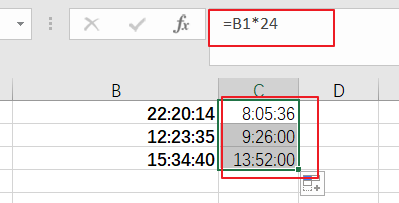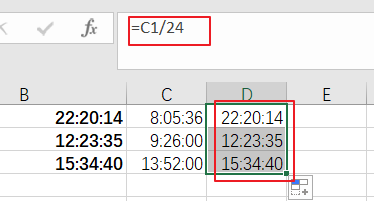# How to Convert Time to Decimal Hours in Excel

This post will guide you how to convert Excel time to Decimal hours with a formula in Excel. How do I convert decimal hours to times in Excel 2013/2016.

## Convert Time to Decimal Hours

Assuming that you have a list of data in range B1:B4, in which contain time values, and you want to convert it into decimal hours. How to accomplish it. You just need multiply by 24 for each cell, so just using the following formula:

=B1*24## Convert Decimal Hours to Time

If you want to convert a decimal hour to Excel Time, just simply divide by 24. So you can use the following formula:

=C1/24

Type this formula into a blank cell and press Enter key on your keyboard, then drag the AutoFill handle down to other cells to apply this formula.